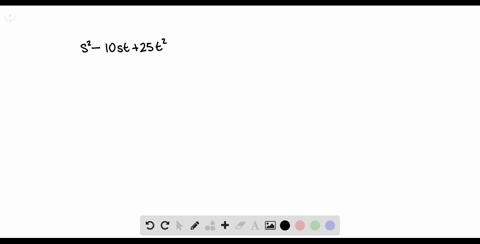Enroll in one of our FREE online STEM summer camps. Space is limited so join now!View Summer Courses### Factor each polynomial. If a polynomial cannot be…

00:51Other Schools

Need more help? Fill out this quick form to get professional live tutoring.

Get live tutoring
Problem 25

Factor each polynomial. If a polynomial cannot be factored, write prime. Factor out the greatest common factor as necessary.
$$z^{2}+14 z y+49 y^{2}$$

$z^{2}+14 z y+49 y^{2}=(z+7 y)^{2}$

## Discussion

You must be signed in to discuss.

## Video Transcript

Rast affected this trying O'Neill here. It has a leading coefficient of one. That means we can look at our 49. So we want to find two factors that multiply together to be 49 and add together to be 14. So that would be seven and seven. So this tells us this can be factored into said plus satellite and said Plus seven, Why? And since both terms, all right, both factors are the same. We can actually write this as that cost seven. Why all squared?